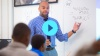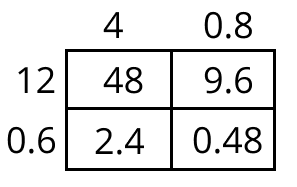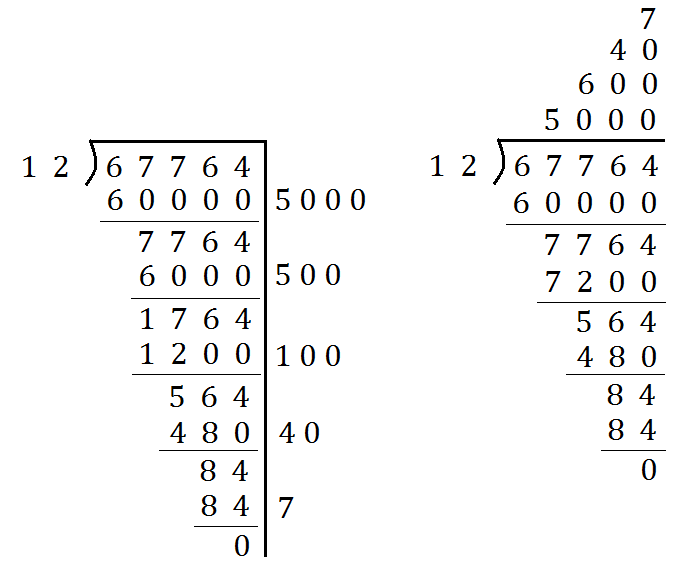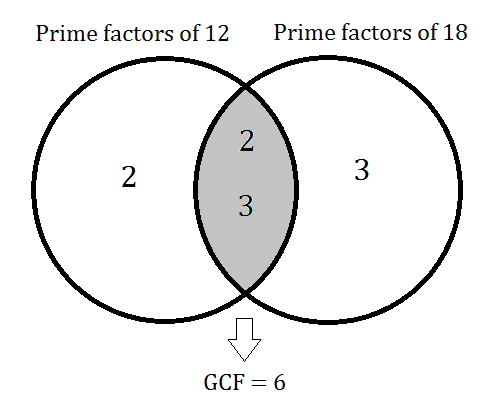# Multi-Digit and Fraction Computation

Students extend their understanding of multiplication and division to divide fractions by fractions, and develop fluency with whole number and decimal operations.

Math

Unit 3

## Unit Summary

In Unit 3, 6th grade students focus on the number system, extending their understanding of multiplication and division to include fraction division, and developing fluency with whole number and decimal operations. Throughout the unit, students work toward developing and understanding efficient algorithms. By examining the structure of concrete models and patterns that emerge from these structures, students make sense of concepts such as multiplying by a reciprocal of a fraction when dividing or using long division as a shorthand to partial quotients (MP.8). With these efficient computation algorithms, students solve and interpret real-world problems, including rate applications from Unit 2. Throughout this unit, students will develop, practice, and demonstrate fluency with decimal operations; however, practice and demonstration opportunities should continue throughout the year with the goal of fluency by the end of the year. Several opportunities are already built into future units, such as the unit on Expressions and the unit on Equations, but additional opportunities need to be planned for and included. See our Procedural Skill and Fluency Guide for additional information and strategy and activity suggestions.

Throughout elementary grades, students developed their understanding of the base-ten system. They found sums and products and quotients by using concrete models, place value, properties of operations, and the relationships between operations. Intentionally, students did not learn a standard algorithm until they had the conceptual understanding to back it up. Some of these strategies are revisited in this unit in order to ensure that students firmly understand the reasoning behind an algorithm, rather than using it without understanding.

Once students have completed their study of the positive number system of fractions, decimals, and whole numbers, they will investigate the numbers to the left of 0 on the number line, or negative rational numbers, in Unit 4. In 7th Grade Math, students will learn how to compute with all rational numbers, including negatives, and in 8th Grade Math and high school, students learn about irrational numbers, rounding out their study of the real number system.

Pacing: 20 instructional days (17 lessons, 2 flex days, 1 assessment day)

Fishtank Plus for Math

Unlock features to optimize your prep time, plan engaging lessons, and monitor student progress.## Assessment

The following assessments accompany Unit 3.

### Pre-Unit

Have students complete the Pre-Unit Assessment and Pre-Unit Student Self-Assessment before starting the unit. Use the Pre-Unit Assessment Analysis Guide to identify gaps in foundational understanding and map out a plan for learning acceleration throughout the unit.

### Mid-Unit

Have students complete the Mid-Unit Assessment.

### Post-Unit

Use the resources below to assess student understanding of the unit content and action plan for future units.

Expanded Assessment Package

Use student data to drive your planning with an expanded suite of unit assessments to help gauge students’ facility with foundational skills and concepts, as well as their progress with unit content.

## Unit Prep

### Intellectual Prep

Unit Launch

Prepare to teach this unit by immersing yourself in the standards, big ideas, and connections to prior and future content. Unit Launches include a series of short videos, targeted readings, and opportunities for action planning.#### Internalization of Standards via the Post-Unit Assessment

• Take Post-Unit Assessment. Annotate for:
• Standards that each question aligns to
• Strategies and representations used in daily lessons
• Relationship to Essential Understandings of unit
• Lesson(s) that Assessment points to

#### Internalization of Trajectory of Unit

• Read and annotate the Unit Summary.
• Notice the progression of concepts through the unit using the Lesson Map.
• Essential Understandings
• Connection to Post-Unit Assessment questions
• Identify key opportunities to engage students in academic discourse. Read through our Teacher Tool on Academic Discourse and refer back to it throughout the unit.

#### Unit-Specific Intellectual Prep

 Model Example Partial products Multiply 12.6 and 4.8 using partial products.Partial quotients Divide 67,764 by 12 using partial quotients.Venn Diagram Use a Venn diagram to find the GCF of 12 and 18.### Essential Understandings

• Dividing by a fraction is the same as multiplying by the denominator and dividing by the numerator, or equivalently, multiplying by the reciprocal of the fraction.
• The general approach to dividing with fractions can be applied to real-world problems involving division, such as partitioning into equal groups and finding missing factors.
• Standard algorithms for computing with decimals are efficient strategies to add, subtract, multiply, and divide decimals. These algorithms are rooted in the same concepts as previously learned strategies.
• Every number can be decomposed into a product of prime factors. These prime factors can be used to find greatest common factors and least common multiples between pairs of numbers.

### Vocabulary

composite number

divisor

dividend

greatest common factor (gcf)

least common multiple (lcm)

long division/ standard algorithm for division

prime number

prime factorization

quotient

reciprocal

relatively prime

To see all the vocabulary for Unit 3, view our 6th Grade Vocabulary Glossary.

### Materials

• Optional: Calculators (1 per student) — Calculators can be used to check answers.
• Graph Paper (2-3 sheets per student)
• Markers or crayons (1 set per small group)
• Poster paper (1 per small group)
• Optional: Dominoes cards (1 per small group) — The cards can be printed so the pictures can be cut out and optionally pasted onto a cardstock or a different colored paper background.

To see all the materials needed for this course, view our 6th Grade Course Material Overview.

## Unit Practice

Word Problems and Fluency Activities

Access daily word problem practice and our content-aligned fluency activities created to help students strengthen their application and fluency skills.## Lesson Map

Topic A: Dividing with Fractions

Topic B: Computing with Decimals

Topic C: Applying the Greatest Common Factor and the Least Common Multiple

## Common Core Standards

Key

Major Cluster

Supporting Cluster

### Core Standards

#### The Number System

• 6.NS.A.1 — Interpret and compute quotients of fractions, and solve word problems involving division of fractions by fractions, e.g., by using visual fraction models and equations to represent the problem. For example, create a story context for (2/3) ÷ (3/4) and use a visual fraction model to show the quotient; use the relationship between multiplication and division to explain that (2/3) ÷ (3/4) = 8/9 because 3/4 of 8/9 is 2/3. (In general, (a/b) ÷ (c/d) = ad/bc.) How much chocolate will each person get if 3 people share 1/2 lb of chocolate equally? How many 3/4-cup servings are in 2/3 of a cup of yogurt? How wide is a rectangular strip of land with length 3/4 mi and area 1/2 square mi?
• 6.NS.B.2 — Fluently divide multi-digit numbers using the standard algorithm.
• 6.NS.B.3 — Fluently add, subtract, multiply, and divide multi-digit decimals using the standard algorithm for each operation.
• 6.NS.B.4 — Find the greatest common factor of two whole numbers less than or equal to 100 and the least common multiple of two whole numbers less than or equal to 12. Use the distributive property to express a sum of two whole numbers 1—100 with a common factor as a multiple of a sum of two whole numbers with no common factor. For example, express 36 + 8 as 4 (9 + 2).

• 4.NBT.B.6
• 5.NBT.A.2
• 5.NBT.B.5
• 5.NBT.B.6
• 5.NBT.B.7

• 5.NF.B.6
• 5.NF.B.7

• 3.OA.A.3
• 4.OA.B.4

• 7.NS.A.1
• 7.NS.A.2
• 7.NS.A.3

### Standards for Mathematical Practice

• CCSS.MATH.PRACTICE.MP1 — Make sense of problems and persevere in solving them.

• CCSS.MATH.PRACTICE.MP2 — Reason abstractly and quantitatively.

• CCSS.MATH.PRACTICE.MP3 — Construct viable arguments and critique the reasoning of others.

• CCSS.MATH.PRACTICE.MP4 — Model with mathematics.

• CCSS.MATH.PRACTICE.MP5 — Use appropriate tools strategically.

• CCSS.MATH.PRACTICE.MP6 — Attend to precision.

• CCSS.MATH.PRACTICE.MP7 — Look for and make use of structure.

• CCSS.MATH.PRACTICE.MP8 — Look for and express regularity in repeated reasoning.

Unit 2

Unit Rates and Percent

Unit 4

Rational Numbers

## Request a Demo

See all of the features of Fishtank in action and begin the conversation about adoption.

Yes

No

### Any other information you would like to provide about your school?